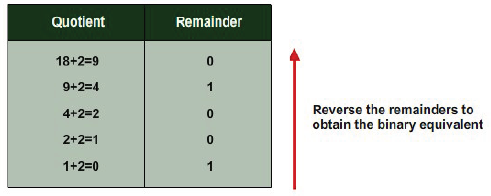#### Decimal Base to Binary Base conversion

Problem Definition: Decimal to binary base conversion.

Problem Analysis: A number with base ‘n’ will contain digits from 0 to n1.

Hence decimal base system is 10.

We use in our normal containing digits from 09.

For example, 922 10 is a number with base 10.

A binary number system has a base 2.

Hence it uses just two numbers i.e. 0 and 1.

The computer system stores information in the binary number system.

The conversion of decimal number to binary number is done by dividing the decimal number by

2 repeatedly and by accumulating the remainders obtained, till the quotient becomes zero.

The reverse of the accumulated remainders is the binary, equivalent the decimal number.

Solving by Example: Consider the decimal number (18)10Hence (18)10 equivalent to (10010)2.

Algorithm Definition

Step 1: Start

Step 2: Read the decimal number n that has to be converted to binary.

Step 3: Initialize bin to zero.

Step 4: Initialize i to zero.

Step 5: Repeat the following steps unit n is greater than zero.

(i) Divide n by 2

(ii) Store the quotient to number

(iii) Add the value (10*Remainder) to the value i bin:

(iv) increment i by 1

Step 6: Print the value of bin.

Step 7: Stop